## write the equation of the line for the following table of values. x. -3 -5 -7 -9 -11

Question

write the equation of the line for the following table of values.

x. -3 -5 -7 -9 -11

y. -16 -26 -36 -46 -56

Plz helppp 35 points:)​

in progress 0
5 months 2021-08-25T18:23:58+00:00 1 Answers 0 views 0

We conclude that the equation of the line is:

•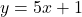Step-by-step explanation:

The slope-intercept form of the line equation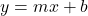where

• m is the slope
• b is the y-intercept

Given the data table

x      -3 -5 -7 -9 -11

y      -16 -26 -36 -46 -56

From the table taking two points

• (-3, -16)
• (-5, -26)

Determining the slope between (-3, -16) and (-5, -26)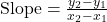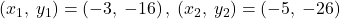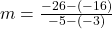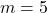Thus, the slope of the line is:  m = 5

substituting m = 5 and (-3, -16) in the slope-intercept form of the line equation  to determine the y-intercept b-16 = 5(-3) + b

-16 = -15 + b

b = -16+15

b = 1

Thus, the y-intercept b = 1

now substituting m = 5 and b = 1 in the slope-intercept form of the line equationTherefore, we conclude that the equation of the line is:

•